# Depth-First Search (DFS) and Breadth-First Search (BFS) for noobs

Things we will be talking about in the article:

• What is DFS
• Example of DFS
• Applications of DFS
• Analysis of DFS
• What is BFS?
• Example of BFS
• Applications of BFS
• Analysis of BFS
• Summary

DFS and BFS are Graph traversal methods. So, what does graph traversal mean? Essentially, the thought is that we’ll be traversing around the graph starting with one vertex then onto the next and finding properties of their intertwined connections. DFS and BFS are two of the most common algorithms used for Graph Traversals. While both of them help us in traversal, they work completely differently. Let us begin with DFS.

# Depth-First Search or DFS:

The name is kind of self-explanatory, it hints at the idea of ‘going deep’ and ‘exploring’. The idea is that we start from any vertex of our choice, we visit it and then ‘explore’ it to locate its adjacent vertices. Once we decide to visit one of its adjacent vertices, we explore it until we reach the end of it and it cannot be explored any further. If we don’t reach the desired destination, we ‘back-track’ and traverse an alternate way.

By this point, you must’ve gotten a rough idea of what DFS is. Now we’ll proceed with an example to make the concept crystal clear in your mind.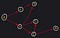Directed Graph Taken as an Example For Better Understanding

As stated above, we can start with any vertex of our liking, I chose to start with ‘A’ for simple and better understanding. Example here is a directed graph, which means we can traverse from one vertex to another only according to the directions of the arrow.

For DFS we are going to start from ‘A’, choose one of its adjacent vertices, and explore it completely and if ‘B’ is not located, we backtrack to ‘A’ and go down another path(Explore another adjacent vertex of ’A’)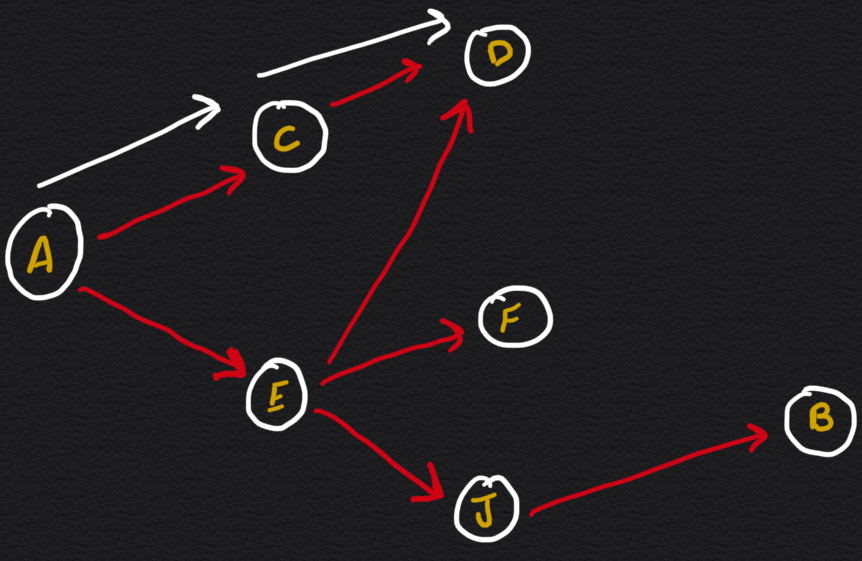Exploring the path of the first adjacent vertex

I chose to visit vertex ‘C’ from ‘A’, it has come to our notice that this is not the end because it further leads to another vertex ‘D’, so we further explore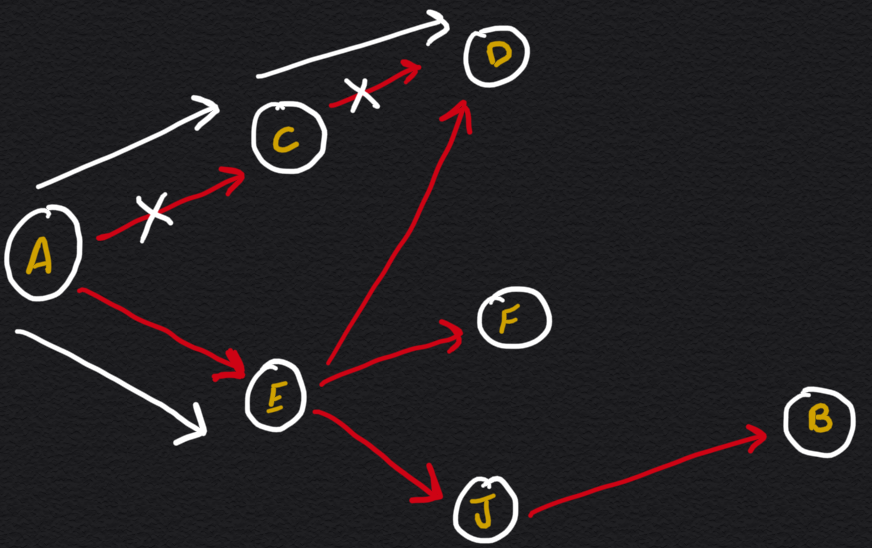Going down the path of the second adjacent vertex of source node ‘A’

As we see in the above image, ACD doesn’t lead to ‘B’ so we have to backtrack to ‘A’. Now, from ‘A’, we head to thesecond adjacent node/vertex.

NOTE: we can choose which adjacent vertex to explore first in any order we like, for simplicity and better understanding I decided to go TOP to BOTTOM.

Now, heading to ‘E’, as we can see, it has 3 adjacent vertices, we will go down the second path i.e. to F.

Once again, similar to the previous path, we reach a dead-end that does not lead to ‘B’.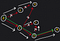Finally! we reach ‘B’, the destination.

we’ll backtrack to ‘E’ and head to ‘J’ and once we explore it further we stumble upon ‘B’, finally!

This should give you a clear understanding of how DFS works. You explore a path until you either reach the desired destination or a dead-end. If you hit a dead-end, you trackback to the previous node and explore the other remaining adjacent vertices.

You might have noticed that we need to follow the same procedure for each of the vertices we visit, so it’s kind of obvious that we will need to use recursion to implement DFS.

# Applications of DFS

In this specific example, we’re using DFS algorithms to determine whether it’s possible to reach from one node to another but it has many more applications than this, I’ll state some of them below

1) To get the minimum spanning tree for an unweighted graph, DFS traversal can be performed.

2) Detecting cycle in a graph: A graph has a cycle if and just on the off chance that we see a back edge during DFS. So we can run DFS for the graph and check for back edges.

3) Path Finding: We can utilize the DFS algorithm to find a path between the two given vertices A and B like we did in the example above.

i) Call DFS(G, A) with ‘A’ as the start vertex.

ii) We use data structure stack, ‘S’ to keep track of the path between the ‘A’ and the current vertex.

iii) When vertex ‘B’ is encountered, return the path as the contents of the stack

4) Finding Strongly Connected Components of a graph: A directed graph is called strongly connected if there is a path from each vertex in the graph to every other vertex.

5) Solving puzzles with only one solution: for example, mazes.

DFS can be utilized to find all solutions to a maze by including only the nodes on the current path in the set of visited nodes.

NOTE: There are many more applications of DFS than I have covered here in the article. I chose these one’s just to give you a clear idea of what all problems does DFS help us solve.

# Analysis of DFS

Since we’re traversing through each adjacent vertex of the node and will be ignoring the vertices that have already been visited once, this gives us a runtime of O(V + E).

Now, you must be wondering what ‘V+E’ is and where it came from so, here’s a quick explanation

V — total number of Vertices

E — Total number of Edges

Every vertex can have an ’n’ number of edges.

It might appear to be that one might be persuaded that it is V•E rather than V + E, we should consider what “V•E” implies precisely.

For the runtime to be V•E, it would imply that, for every vertex, we need to look at all the edges in the graph whether or not those specific edges are associated with that particular vertex or are adjacent to that vertex.

While, then again, “V + E” implies that for every vertex, we just gander at the count of all the edges that are adjacent to that vertex. O(V + E) is the space we take up for the contiguousness/adjacency list. Every vertex has various edges and in the worst-case scenario, if we somehow managed to apply DFS on every vertex, O(V) work would’ve been done alongside exploiting every one of the edges of all the vertices, which comes out to be O(E). Whenever we’ve taken a gander at all ‘V’ vertices, we would have likewise taken a gander at an aggregate of E edges. Subsequently, it’s (V + E).

# Breadth-First Search or BFS

Now, let's have a loot at BFS to see how it differs.

As the name suggests, BFS or Breadth-First Seach can be looked at as ‘going wide’ or having the ‘bird’s eye view’ approach. What that fundamentally implies is that as opposed to going deep and exploring one path like DFS, BFS moves towards its objective each adjacent vertex at a time.

Let’s use the previous example again to better understand this concept:Same graph as before

Instead of completely exploring one adjacent vertex of ‘A’ till the end, in BFS we will visit each adjacent vertex of ‘A’ and then visit and explore each of them until we finally reach ‘B’.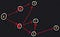we look at its neighbours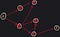Then look at its neighbours’ neighbours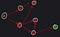Then eventually we locate ‘B’

Perceive how distinctly DFS and BFS act? While I believe that DFS prefers to go head-on, BFS moves slowly, notices its surroundings and then move one step at a time.

One question might arise in your mind that: “which neighbour to visit first out of all of A’s neighbours?”

To solve this we could use the queue’s FIFO (first-in-first-out) property where we pop the primary vertex of the queue, then add its unvisited neighbours to the queue itself, and afterwards, proceed with this interaction until that queue is either vacant or that the vertex that we are about to add to the queue is the one we’ve been searching for.

# Application of BFS

1) Briefest Path and Minimum Spanning Tree for unweighted graphs: for unweighted graphs, the shortest path is the path with the least number of edges. With the BFS approach, we can reach the vertex from a given source node using the minimum number of edges. For unweighted graphs, all spanning trees are Minimum Spanning Tree and either DFS or BFS traversal can be utilised for finding the spanning tree.

2) Peer to Peer Networks: In Peer-to-Peer Networks like BitTorrent, BFS is used to find all adjacent nodes.

3) Crawlers in Search Engines: Crawlers build an index using Breadth First approach. The thought is to begin from the source page and follow all connections from the source and continue to do the same. Profundity First Traversal can likewise be utilized for crawlers, however the benefit with Breadth-First Traversal is, profundity or levels of the fabricated tree can be restricted.

4) GPS: BFS can be utilised in finding all neighbouring locations.

5) Broadcasting in Network: In networks, a broadcasted message packet follows BFS to reach/cover all nodes.

6) Cycle detection in an undirected graph: In undirected graphs, either BFS or DFS can be used to detect cycles. We can use BFS to detect a cycle in directed graphs too.

7) To test if a graph is Bipartite: either BFS or DFS both can be used for this.

8) Path Finding: can utilise either BFS or DFS Traversal to find if there is a path between two vertices in a graph.

9) Finding all nodes adjacent to one specific node: can utilise either BFS or DFS Traversal to find all adjacent nodes from a given node.

Many algorithms like Prim’s Minimum Spanning Tree and Dijkstra’s Single Source Shortest Path use a similar approach to BFS.

I’ve not displayed every application of BFS here. There are a lot more applications as BFS is one of the main algorithms for Graph Traversal.

# Analysis of BFS

It might appear as though BFS is more slow. However, in the event that you take a gander at the representations of both DFS and BFS, you’ll see that they really have equivalent runtimes.

The queue guarantees that probably every vertex will be visited until it arrives at the desired node. So that implies at the worst-case scenario, BFS will likewise take a gander at all of the vertices and edges.

While BFS might appear to be a bit slow as compared to DFS, it’s really considered quicker since, in such a case that we were to execute them on bigger diagrams, you’ll see that DFS burns through a great deal of time traversing down long routes that's at last off-base. Truth be told, BFS is regularly utilized in calculations to decide the quickest path starting with one vertex then onto the next

Now, since the runtimes for both are similar, O(V + E) is the runtime of BFS and because of the utilization of queue that can hold all things considered V vertices, O(V) is the space complexity for it.

# Summary

• The purpose of both, Depth-First Search and Breadth-First Search, is graph traversal.
• DFS explores a path until it has visited all the neighbours and the neighbours’ neighbours to find the desired node, while BFS utilises the ‘Bird's eye view’ approach of going wide through adjacent vertices to find the desired node.
• DFS is implemented via Stack while BFS is implemented via Queue data structure.
• O(V + E), this is the runtime for both DFS and BFS. Similarly, space complexity is O(V).

Full Stack Web Developer | Programmer | Finance and technology fascinate me.

## More from Anmol Arora

Full Stack Web Developer | Programmer | Finance and technology fascinate me.